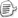#include "stdio.h"
#define SWAP_TWO_INT(a, b) \
a^=b; b^=a; a^=b;
class CBinaryHeap
{
public:
CBinaryHeap(int iSize = 100);
~CBinaryHeap();

//Return 0 means failed.
int Enqueue(int iVal);
int Dequeue(int &iVal);
int GetMin(int &iVal);

#ifdef _DEBUG
void PrintQueue();
#endif

protected:
int *m_pData;
int m_iSize;
int m_iAmount;
};

CBinaryHeap::CBinaryHeap(int iSize)
{
m_pData = new int[iSize];
m_iSize = iSize;
m_iAmount = 0;
}

CBinaryHeap::~CBinaryHeap()
{
delete[] m_pData;
}

#ifdef _DEBUG
int CBinaryHeap::Enqueue(int iVal)
{
if(m_iAmount==m_iSize)
return 0;

//Put this value to the end of the array.
m_pData[m_iAmount] = iVal;
++m_iAmount;

int iIndex = m_iAmount - 1;
while(m_pData[iIndex] < m_pData[(iIndex-1)/2])
{
//Swap the two value
SWAP_TWO_INT(m_pData[iIndex], m_pData[(iIndex-1)/2])
iIndex = (iIndex-1)/2;
}

return 1;
}
#endif

int CBinaryHeap::Dequeue(int &iVal)
{
if(m_iAmount==0)
return 0;

iVal = m_pData;
int iIndex = 0;
while (iIndex*2<m_iAmount)
{
int iLeft = (iIndex*2+1 < m_iAmount)?(iIndex*2+1):0;
int iRight = (iIndex*2+2 < m_iAmount)?(iIndex*2+2):0;
if(iLeft && iRight) // Both left and right exists.
{
if(m_pData[iLeft]<m_pData[iRight])
{
SWAP_TWO_INT(m_pData[iIndex], m_pData[iLeft])
iIndex = iLeft;
}
else
{
SWAP_TWO_INT(m_pData[iIndex], m_pData[iRight])
iIndex = iRight;
}
}
else if(iLeft) //The iRight must be 0
{
SWAP_TWO_INT(m_pData[iIndex], m_pData[iLeft])
iIndex = iLeft;
break;
}
else
{
break;
}
}

//Move the last element to the blank position.
//Of course, if it is the blank one, forget it.
if(iIndex!=m_iAmount-1)
{
m_pData[iIndex] = m_pData[m_iAmount-1];

//Try to move this element to the top as high as possible.
while(m_pData[iIndex] < m_pData[(iIndex-1)/2])
{
//Swap the two value
SWAP_TWO_INT(m_pData[iIndex], m_pData[(iIndex-1)/2])
iIndex = (iIndex-1)/2;
}
}

--m_iAmount;

return 1;
}

int CBinaryHeap::GetMin(int &iVal)
{
if(m_iAmount==0)
return 0;
iVal = m_pData;
return 1;
}

void CBinaryHeap::PrintQueue()
{
int i;
for(i=0; i<m_iAmount; i++)
{
printf("%d ", m_pData[i]);
}
printf("\n");
}

int main(int argc, char* argv[])
{
CBinaryHeap bh;
bh.Enqueue(4);
bh.Enqueue(1);
bh.Enqueue(3);
bh.Enqueue(2);
bh.Enqueue(6);
bh.Enqueue(5);
#ifdef _DEBUG
bh.PrintQueue();
#endif

int iVal;
bh.Dequeue(iVal);
bh.Dequeue(iVal);
#ifdef _DEBUG
bh.PrintQueue();
#endif
return 0;
}posted on 2010-06-28 15:08  chao_yu  阅读(7987)  评论(1编辑  收藏  举报